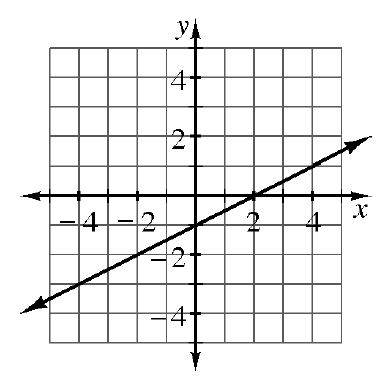### Home > CC3MN > Chapter 4 > Lesson 4.1.3 > Problem4-28

4-28.Another one of the connections in your Representations of Patterns Web is graph $→$ table. In Chapters 1 through 3, you developed tools to find a table from a graph. Consider this connection as you complete the table below. The table is based on the graph at right.

 IN (x) OUT (y) $-3$ $-2$ $-1$ $0$ $1$ $2$ $3$

Use the eTool below to find the y coordinates on the graph and complete the table.
Click the link at right for the full version of the eTool:   4-28 HW eTool (Desmos)